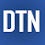## Pages

### Gradient Boosting Regression Example with GBM in R

The gbm package provides the extended implementation of Adaboost and Friedman's gradient boosting machines algorithms. In this tutorial, we'll learn how to use the gbm model for regression in R. The post covers:
1. Preparing data
2. Using the gbm method
3. Using the gbm with a caret

```library(gbm)
library(caret)```

Preparing data

In this tutorial, we'll use Boston housing dataset as regression data.
We'll load the dataset it is in MASS packages.

`boston = MASS::Boston`

You can check the data content.

```str(boston)
'data.frame': 506 obs. of  14 variables:
\$ crim   : num  0.00632 0.02731 0.02729 0.03237 0.06905 ...
\$ zn     : num  18 0 0 0 0 0 12.5 12.5 12.5 12.5 ...
\$ indus  : num  2.31 7.07 7.07 2.18 2.18 2.18 7.87 7.87 7.87 7.87 ...
\$ chas   : int  0 0 0 0 0 0 0 0 0 0 ...
\$ nox    : num  0.538 0.469 0.469 0.458 0.458 0.458 0.524 0.524 0.524 0.524 ...
\$ rm     : num  6.58 6.42 7.18 7 7.15 ...
\$ age    : num  65.2 78.9 61.1 45.8 54.2 58.7 66.6 96.1 100 85.9 ...
\$ dis    : num  4.09 4.97 4.97 6.06 6.06 ...
\$ rad    : int  1 2 2 3 3 3 5 5 5 5 ...
\$ tax    : num  296 242 242 222 222 222 311 311 311 311 ...
\$ ptratio: num  15.3 17.8 17.8 18.7 18.7 18.7 15.2 15.2 15.2 15.2 ...
\$ black  : num  397 397 393 395 397 ...
\$ lstat  : num  4.98 9.14 4.03 2.94 5.33 ...
\$ medv   : num  24 21.6 34.7 33.4 36.2 28.7 22.9 27.1 16.5 18.9 ...```

Here, 'medv' is a target - y output and the others are the features - x input data.

Next, we'll split the dataset into the train and test parts.

```indexes = createDataPartition(boston\$medv, p = .90, list = F)
train = boston[indexes, ]
test = boston[-indexes, ]```

Using the gbm method

We'll define the gbm model with gaussiann distribution and I set the other parameters as below. And, we'll include train data to fit the model.

```model_gbm = gbm(train\$medv ~.,
data = train,
distribution = "gaussian",
cv.folds = 10,
shrinkage = .01,
n.minobsinnode = 10,
n.trees = 500)
```
```print(model_gbm)
gbm(formula = train\$medv ~ ., distribution = "gaussian", data = train,
n.trees = 500, n.minobsinnode = 10, shrinkage = 0.01, cv.folds = 10)
A gradient boosted model with gaussian loss function.
500 iterations were performed.
The best cross-validation iteration was 500.
There were 13 predictors of which 9 had non-zero influence.```

Next, we'll predict test data and visialize the result in a plot.

```test_x = test[, -14]
test_y = test[, 14]

pred_y = predict.gbm(model_gbm, test_x)
x_ax = 1:length(pred_y)
plot(x_ax, test_y, col="blue", pch=20, cex=.9)
lines(x_ax, pred_y, col="red", pch=20, cex=.9) ```

Using the gbm with caret

We can also use gbm with caret train method.

```tc = trainControl(method = "cv", number=10)
model = train(medv ~., data=train, method="gbm", trControl=tc)```

We'll predict test data and visualize in a plot.

```pred_y = predict(model, test_x)
x_ax = 1:length(pred_y)
plot(x_ax, test_y, col="blue", pch=20, cex=.9)
lines(x_ax, pred_y, col="red", pch=20, cex=.9)```

In this post, we've briefly learned how to use gradient boosting method with gbm package for regression data. Thank you for reading.
The full source code is listed below.
``````
library(gbm)
library(caret)

boston = MASS::Boston
str(boston)
indexes = createDataPartition(boston\$medv, p = .90, list = F)
train = boston[indexes, ]
test = boston[-indexes, ]

model_gbm = gbm(train\$medv ~.,
data = train,
distribution = "gaussian",
cv.folds = 10,
shrinkage = .01,
n.minobsinnode = 10,
n.trees = 500)

print(model_gbm)

test_x = test[, -14]
test_y = test[, 14]

pred_y = predict.gbm(model_gbm, test_x)
caret::R2(test_y, pred_y)
RMSE(test_y, pred_y)

x_ax = 1:length(pred_y)
plot(x_ax, test_y, col="blue", pch=20, cex=.9)
lines(x_ax, pred_y, col="red", pch=20, cex=.9)

# with caret method
tc = trainControl(method = "cv", number=10)
model = train(medv ~., data=train, method="gbm", trControl=tc)
print(model)
pred_y = predict(model, test_x)
RMSE(test_y, pred_y)

x_ax = 1:length(pred_y)
plot(x_ax, test_y, col="blue", pch=20, cex=.9)
lines(x_ax, pred_y, col="red", pch=20, cex=.9)

``````

1.1.